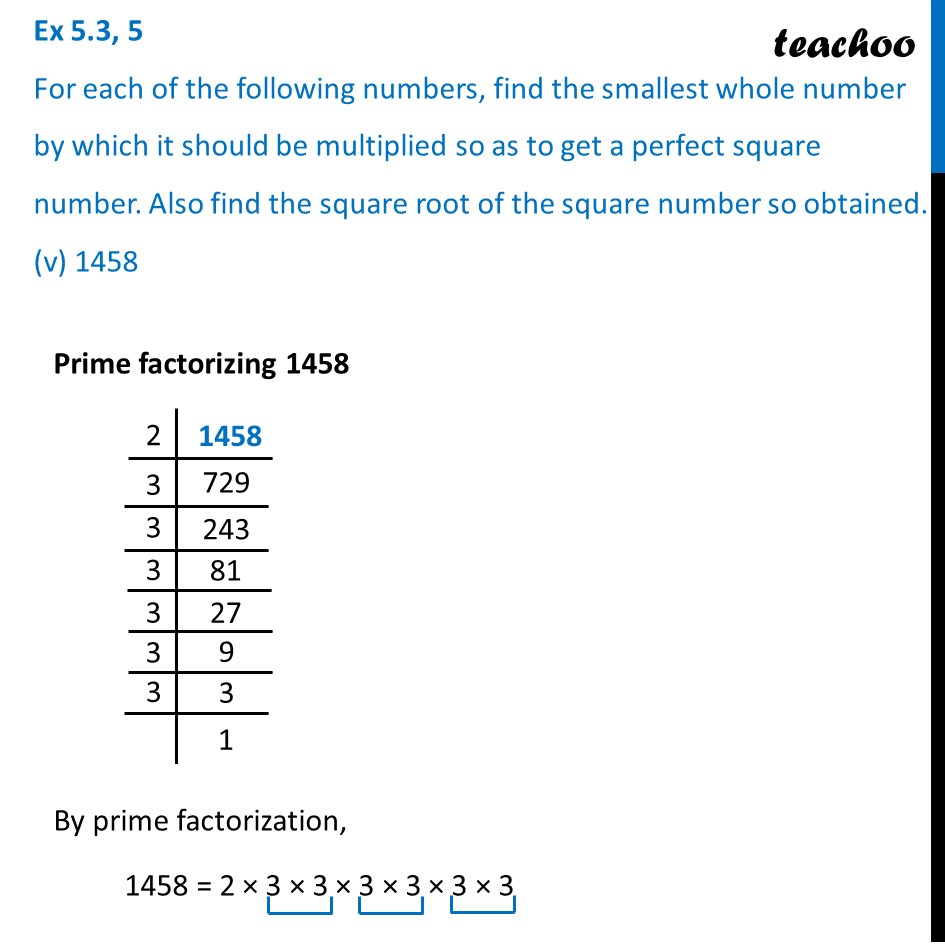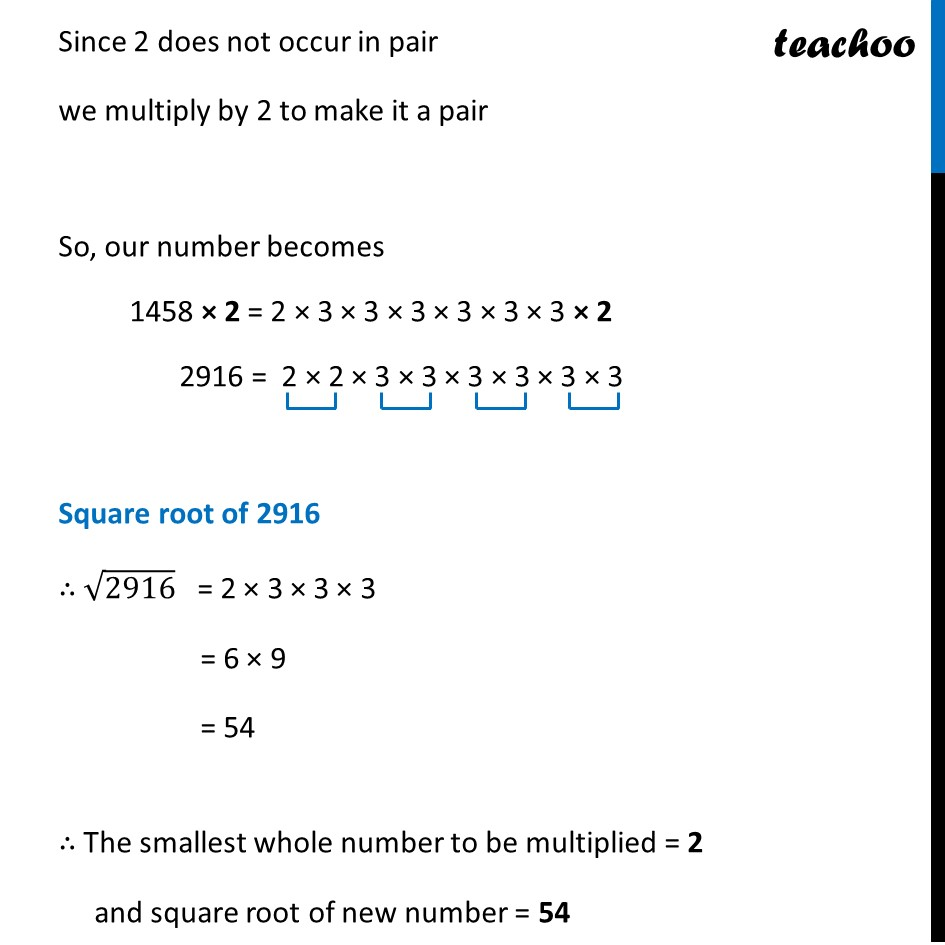Ex 5.3

Chapter 5 Class 8 Squares and Square Roots
Serial order wiseLearn in your speed, with individual attention - Teachoo Maths 1-on-1 Class

### Transcript

Ex 5.3, 5 For each of the following numbers, find the smallest whole number by which it should be multiplied so as to get a perfect square number. Also find the square root of the square number so obtained. (v) 1458 Prime factorizing 1458 By prime factorization, 1458 = 2 × 3 × 3 × 3 × 3 × 3 × 3 Since 2 does not occur in pair we multiply by 2 to make it a pair So, our number becomes 1458 × 2 = 2 × 3 × 3 × 3 × 3 × 3 × 3 × 2 2916 = 2 × 2 × 3 × 3 × 3 × 3 × 3 × 3 Square root of 2916 ∴ √2916 = 2 × 3 × 3 × 3 = 6 × 9 = 54 ∴ The smallest whole number to be multiplied = 2 and square root of new number = 54